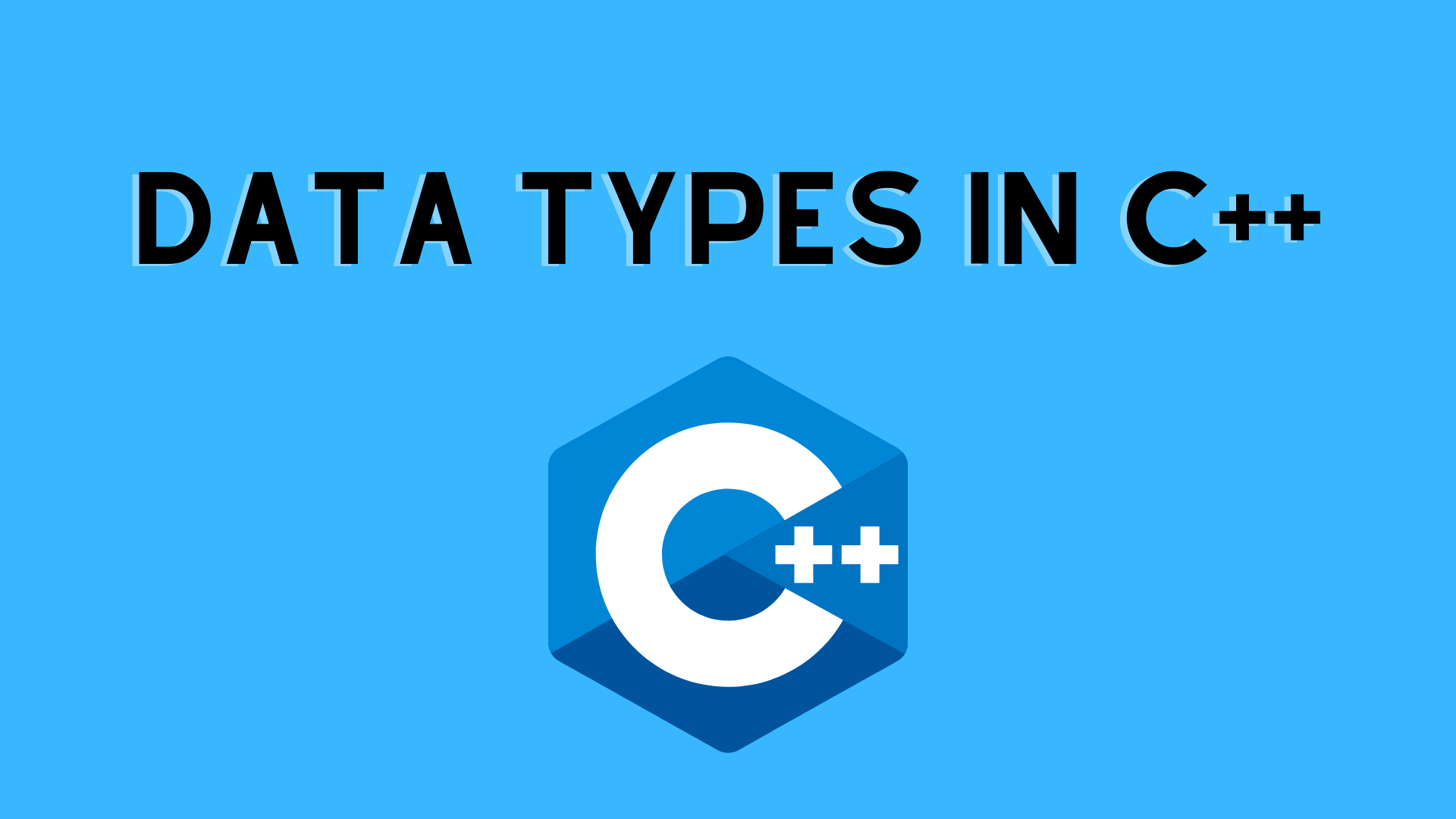## Data Types in C++

Data types in C/C++ are used in the declaration of all variables to limit the type of data that can be stored. As a result, data types are used to inform variables about the types of data they can store. When a variable is declared in C++, the compiler allocates memory for it based on the data type that it is declared with. Each sort of data necessitates a particular quantity of memory.

In C++, data types are separated into three categories:

• 1. Type of Primitive Data
• 2. Data Types Derived from Other Data(Derived data type)
• 3. User-Defined Data Types or Abstract Data Types
1. Primitive Data Types: These data types are built-in or preset data types that the user can declare variables with directly. For instance, int, char, float, bool, and so on. The following primitive data types are available in C++:
• Integer
• Character
• Floating Point Boolean
• Floating Points in Twos
• Wide Character
• Valueless or Void

2. Derived Data Types: Derived Data Types are data types that are derived from primitive or built-in datatypes.

There are four different varieties of these:

• Function
• Array
• Pointer
• Reference

3. User-Defined Data Types: These are data types that are defined by the user. As an example, in C++, defining a class or a structure. The following user-defined datatypes are available in C++:

• Class
• Structure
• Union
• Enumeration
Defining a typedef DataType

4. Modifiers in Datatypes: Datatype modifiers are used with built-in data types to change the length of data a data type can carry, as the name implies.

• Signed
• Unsigned
• Short
• Long
```// C++ program to sizes of data types
#include<iostream>
using namespace std;

int main()
{
cout << "Size of char : " << sizeof(char)
<< " byte" << endl;
cout << "Size of int : " << sizeof(int)
<< " bytes" << endl;
cout << "Size of short int : " << sizeof(short int)
<< " bytes" << endl;
cout << "Size of long int : " << sizeof(long int)
<< " bytes" << endl;
cout << "Size of signed long int : " << sizeof(signed long int)
<< " bytes" << endl;
cout << "Size of unsigned long int : " << sizeof(unsigned long int)
<< " bytes" << endl;
cout << "Size of float : " << sizeof(float)
<< " bytes" <<endl;
cout << "Size of double : " << sizeof(double)
<< " bytes" << endl;
cout << "Size of wchar_t : " << sizeof(wchar_t)
<< " bytes" <<endl;

return 0;
}
```

Output:

Size of int : 4 bytes
Size of short int : 2 bytes
Size of long int : 8 bytes
Size of signed long int : 8 bytes
Size of unsigned long int : 8 bytes
Size of float : 4 bytes
Size of double : 8 bytes
Size of wchar_t : 4 bytes

You may like:

Introduction to C++ Language

Features of C++ | C++ Tutorial